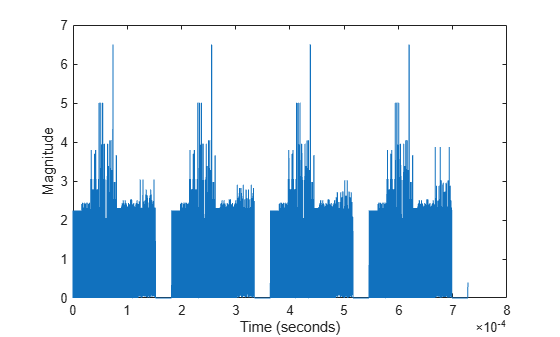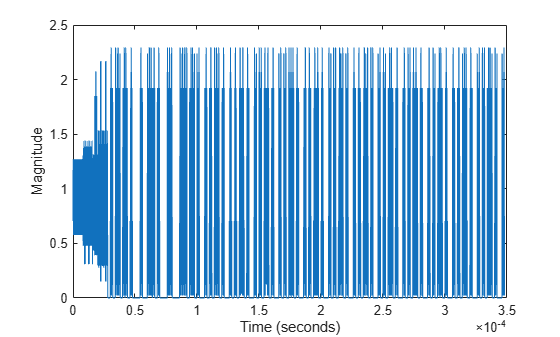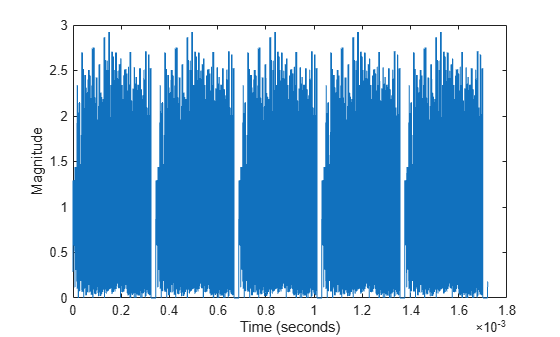## Waveform Generation

After you create the necessary configuration objects described in Create Configuration Objects, you can use the objects to generate the desired WLAN format waveform.

The IEEE® 802.11™ standards define a physical layer conformance procedure (PLCP) protocol data unit (PPDU) as the transmission unit at the physical layer. For a detailed description of the PPDU field structures for each transmission format, see WLAN PPDU Structure.

HE Format PPDU

In HE, there are four transmission modes supported: single user, single user extended range, trigger-based, and multi-user.DMG Format PPDU

In DMG, there are three physical layer (PHY) modulation schemes supported: control, single carrier, and OFDM.S1G Format PPDU

In S1G, there are three transmission modes: S1G_LONG, S1G_SHORT, and S1G_1M. Each transmission mode has a specific PPDU preamble structure.VHT, HT, and non-HT Format PPDUs

The VHT, HT, and non-HT PPDU formats consist of preamble and data fields.Use WLAN Toolbox™ functions to generate a full PPDU waveform or individual PPDU field waveforms.

Generate a full PPDU waveform using the `wlanWaveformGenerator` function to populate all PPDU fields (preamble and data) in a single call. `wlanWaveformGenerator` accepts a bit stream, a format configuration object (`wlanHESUConfig`, `wlanHEMUConfig`, `wlanDMGConfig`, `wlanS1GConfig` `wlanVHTConfig`, `wlanHTConfig`, or `wlanNonHTConfig`) and `Name,Value` pairs to configure the waveform.

### Generate WLAN Waveforms

Generate HE, DMG, S1G, VHT, HT-mixed, and non-HT format waveforms. Vary configuration parameters and plot the waveforms to highlight differences in waveforms and sample rates.

In each section of this example, you:

• Create a format-specific configuration object.

• Create a vector of information bits for the packet data payload. Internally, the `wlanWaveformGeneration` function loops through the bits vector as many times as needed to generate the specified number of packets.

• Generate the format-specific waveform and plot it. For plotting, because no filtering is applied to the waveform and the oversampling rate is 1, set the sampling rate equal to the channel bandwidth.

Generate Single User HE Format Waveform

Create an HE single-user (HE SU) configuration object and waveform. Using `Name,Value` pairs, specify 4 packets and 15 microseconds of idle time. Display the configuration object and inspect its properties and settings.

```cfgHESU = wlanHESUConfig; bits = [1;0;0;1;1]; hesuWaveform = wlanWaveformGenerator(bits,cfgHESU, ... 'NumPackets',4,'IdleTime',15e-6); ```

Plot the single user HE format waveform, scaling the x-axis relative to the channel bandwidth.

```fs = 20e6; % Set sampling frequency equal to the channel bandwidth time = ((0:length(hesuWaveform)-1)/fs)*1e6; plot(time,abs(hesuWaveform)) xlabel ('Time (microseconds)'); ylabel('Magnitude'); ```The plot shows four single user HE format packets, with each packet separated by 15 microseconds of idle time.

Generate Multiuser HE Format Waveform

Create an HE multiuser (HE MU) configuration object and waveform. Using `Name,Value` pairs, specify 3 packets and 30 microseconds of idle time. Display the configuration object and inspect its properties and settings.

```cfgHEMU = wlanHEMUConfig(192); bits = [1;0;0;1;1]; hemuWaveform = wlanWaveformGenerator(bits,cfgHEMU, ... 'NumPackets',3,'IdleTime',30e-6); ```

Plot the multiuser HE format waveform, scaling the x-axis relative to the channel bandwidth.

```fs = 20e6; % Set sampling frequency equal to the channel bandwidth time = ((0:length(hemuWaveform)-1)/fs)*1e6; plot(time,abs(hemuWaveform)) xlabel ('Time (microseconds)'); ylabel('Magnitude'); ```The plot shows three multiuser HE format packets, with each packet separated by 30 microseconds of idle time.

Generate DMG Format Waveform

Create a DMG configuration object and waveform. Using `Name,Value` pairs, assign 13 for the MCS which specifies an OFDM waveform, 4 packets, and 2 microseconds of idle time. Display the configuration object and inspect its properties and settings.

```cfgDMG = wlanDMGConfig('MCS',13); bits = [1;0;0;1;1]; dmgWaveform = wlanWaveformGenerator(bits,cfgDMG, ... 'NumPackets',4,'IdleTime',2e-6); ```

Plot the DMG format waveform, scaling the x-axis relative to the channel bandwidth.

```fs = 2640e6; % Set sampling frequency equal to the channel bandwidth time = ((0:length(dmgWaveform)-1)/fs)*1e6; plot(time,abs(dmgWaveform)) xlabel ('Time (microseconds)'); ylabel('Magnitude'); ```The plot shows four DMG format packets, with each packet separated by 2 microseconds of idle time.

Generate S1G Format Waveform

Create a sub-1-GHz (S1G) configuration object and waveform. Using `Name,Value` pairs, specify 4 MHz channel bandwidth, 3 packets, and 15 microseconds of idle time. Display the configuration object and inspect its properties and settings.

```cfgS1G = wlanS1GConfig('ChannelBandwidth','CBW4'); bits = [1;0;0;1;1]; s1gWaveform = wlanWaveformGenerator(bits,cfgS1G, ... 'NumPackets',3,'IdleTime',15e-6); ```

Plot the S1G format waveform, scaling the x-axis relative to the channel bandwidth.

```fs = 4e6; % Set sampling frequency equal to the channel bandwidth time = ((0:length(s1gWaveform)-1)/fs)*1e6; plot(time,abs(s1gWaveform)) xlabel ('Time (microseconds)'); ylabel('Magnitude'); ```The plot shows three S1G format packets, with each packet separated by 15 microseconds of idle time.

Generate VHT Format Waveform

Create a VHT configuration object and waveform. Using `Name,Value` pairs, specify 5 packets and 20 microseconds of idle time. Display the configuration object and inspect its properties and settings.

```cfgVHT = wlanVHTConfig; bits = [1;0;0;1;1]; vhtWaveform = wlanWaveformGenerator(bits,cfgVHT, ... 'NumPackets',5,'IdleTime',20e-6); ```

Plot the VHT format waveform, scaling the x-axis relative to the channel bandwidth.

```fs = 80e6; % Set sampling frequency equal to the channel bandwidth time = ((0:length(vhtWaveform)-1)/fs)*1e6; plot(time,abs(vhtWaveform)) xlabel ('Time (microseconds)'); ylabel('Magnitude'); ```The plot shows five VHT format packets, with each packet separated by 20 microseconds of idle time.

Generate HT Format Waveform

Create an HT configuration object and waveform. Using `Name,Value` pairs, specify 5 packets and 30 microseconds of idle time. Display the configuration object and inspect its properties and settings.

```cfgHT = wlanHTConfig; bits = [1;0;0;1;1]; htWaveform = wlanWaveformGenerator(bits,cfgHT, ... 'NumPackets',5,'IdleTime',30e-6); ```

Plot the HT format waveform, scaling the x-axis relative to the channel bandwidth.

```fs = 20e6; % Set sampling frequency equal to the channel bandwidth time = ((0:length(htWaveform)-1)/fs)*1e6; plot(time,abs(htWaveform)) xlabel ('Time (microseconds)'); ylabel('Magnitude'); ```The plot shows five HT format packets, with 30 microseconds of idle time separating each packet.

Generate Non-HT Format DSSS Waveform

Create a non-HT configuration object and generate a non-HT format DSSS waveform with a 2 Mbps data rate. Using `Name,Value` pairs, specify 2 packets and 5 microseconds of idle time. Display the configuration object and inspect its properties and settings.

```cfgNonHT = wlanNonHTConfig('Modulation','DSSS','DataRate','2Mbps'); bits = [1;0;0;1;1]; nhtDSSSWaveform = wlanWaveformGenerator(bits,cfgNonHT, ... 'NumPackets',2,'IdleTime',5e-6); ```

Plot the non-HT Format DSSS waveform, scaling the x-axis relative to the channel bandwidth. As specified in IEEE 802.11-2012, Section 17.1.1, the channel bandwidth is 11 MHz for DSSS.

```fs = 11e6; % Set sampling frequency equal to the channel bandwidth time = ((0:length(nhtDSSSWaveform)-1)/fs)*1e6; plot(time,real(nhtDSSSWaveform),'.') xlabel ('Time (microseconds)'); ylabel('Re[nhtDSSSWaveform]'); axis([8190,8200,-1.1,1.1]) ```Sample values in DSSS modulation are –1 or 1. The plot shows the real values for a section of the waveform that includes the tail end of the first packet, the 5 microsecond idle period, and the beginning of the second packet for the non-HT format DSSS modulated waveform.

Generate Non-HT Format OFDM Waveform

Create a non-HT configuration object and waveform. Using `Name,Value` pairs, specify 4 packets and 45 microseconds of idle time. Display the configuration object and inspect its properties and settings.

```cfgNonHT = wlanNonHTConfig; bits = [1;0;0;1;1]; nhtWaveform = wlanWaveformGenerator(bits,cfgNonHT, ... 'NumPackets',4,'IdleTime',45e-6); ```

Plot the non-HT format OFDM waveform, scaling the x-axis relative to the channel bandwidth.

```fs = 20e6; % Set sampling frequency equal to the channel bandwidth time = ((0:length(nhtWaveform)-1)/fs)*1e6; plot(time,abs(nhtWaveform)) xlabel ('Time (microseconds)'); ylabel('Magnitude'); ```The plot shows four non-HT format OFDM modulated packets, with 45 microseconds of idle time separating each packet.

### Waveforms of Individual PPDU Fields

You can also create a VHT, HT, or non-HT PPDU waveform by generating and concatenating waveforms for individual PPDU fields.

Generating individual PPDU field waveforms, enables you to experiment with the individual fields without generating an entire PPDU.